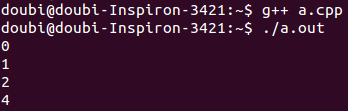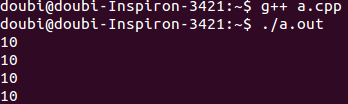C++ vector用法（详解！！函数，实现）c++/c 同时被 2 个专栏收录48 篇文章 12 订阅

１，简述一下vector的基本操作，它的ｓｉｚｅ，capacity（），ｃｌｅａｒ，ｒｅｖｅｒｓｅ，ｒｅｓｅｒｖｅ，

push_back等！！！

２，说说，ｖｅｃｔｏｒ的存储特性，是顺序存储还是如同链表般，如果是顺序存储的话，那么是如何执行

ｅｒａｓｅ，ｉｎｓｅｒｔ等函数，？？？（假如后面的空间不够的话，我们需要合理的算法来重新找出一块

相应的空间吗？？？拷贝，回收吗？？？是不是特别麻烦），如果是链式存储的话，那么它又是如何做到快速

的访问的（通过下标来的）！！！

1 基本操作

(1)头文件#include<vector>.

(2)创建vector对象，vector<int> vec;

(3)尾部插入数字：vec.push_back(a);

(4)使用下标访问元素，cout<<vec<<endl;记住下标是从0开始的。

(5)使用迭代器访问元素.

<span style="font-size:18px;">vector<int>::iterator it;
for(it=vec.begin();it!=vec.end();it++)
cout<<*it<<endl;</span>

(6)插入元素：    vec.insert(vec.begin()+i,a);在第i个元素后面插入a;

(7)删除元素：    vec.erase(vec.begin()+2);删除第3个元素

vec.erase(vec.begin()+i,vec.end()+j);删除区间[i,j-1];区间从0开始

(8)向量大小:vec.size();

(9)清空:vec.clear()　　　//清空之后，vec.size()为０

<span style="font-size:18px;">#include<stdio.h>
#include<vector>
#include<iostream>
using namespace std;
int main()
{
int i=0;
vector<int> vec;
for(i=0; i<10; i++)
{
vec.push_back(i);　　　//10个元素依次进入，结果为10
}

for(unsigned int i=0; i<vec.size(); i++)
{
cout<<"初始化遍历："<<vec[i]<<endl;
}
//结果为：０，１，２，３，４，５，６，７，８，９
vector<int>::iterator it;

for(it = vec.begin(); it!=vec.end(); it++)
{
cout<<"迭代遍历："<<*it<<endl;
}
//结果为：０，１，２，３，４，５，６，７，８，９
vec.insert(vec.begin()+4,0);
//结果为:11
for(unsigned int i=0; i<vec.size(); i++)
{
cout<<"插入遍历："<<vec[i]<<endl;
}
//结果为：０，１，２，３，０，４，５，６，７，８，９
vec.erase(vec.begin()+2);
for(unsigned int i=0; i<vec.size(); i++)
{
cout<<"擦除遍历："<<vec[i]<<endl;
}
//结果为：０，１，３，０，４，５，６，７，８，９
vec.erase(vec.begin()+3,vec.begin()+5);

for(vector<int>::iterator it = vec.begin(); it!=vec.end(); it++)
{
cout<<"迭代遍历："<<*it<<endl;
}
return 0;
}

</span>

2:

vector的元素不仅仅可以使int,double,string,还可以是结构体，但是要注意：结构体要定义为全局的，否则会出错。下面是一段简短的程序代码：

<span style="font-size:18px;">#include<stdio.h>
#include<algorithm>
#include<vector>
#include<iostream>
using namespace std;

typedef struct rect
{
int id;
int length;
int width;

//对于向量元素是结构体的，可在结构体内部定义比较函数，下面按照id,length,width升序排序。
bool operator< (const rect &a)  const
{
if(id!=a.id)
return id<a.id;
else
{
if(length!=a.length)
return length<a.length;
else
return width<a.width;
}
}
}Rect;

int main()
{
vector<Rect> vec;
Rect rect;
rect.id=1;
rect.length=2;
rect.width=3;
vec.push_back(rect);
vector<Rect>::iterator it=vec.begin();
cout<<(*it).id<<' '<<(*it).length<<' '<<(*it).width<<endl;

return 0;

}</span>

3  算法

(1) 使用reverse将元素翻转：需要头文件#include<algorithm>

reverse(vec.begin(),vec.end());将元素翻转(在vector中，如果一个函数中需要两个迭代器，

一般后一个都不包含.)

(2)使用sort排序：需要头文件#include<algorithm>，

sort(vec.begin(),vec.end());(默认是按升序排列,即从小到大).

bool Comp(const int &a,const int &b)
{
return a>b;
}

vector ： C++ STL中的顺序容器，封装数组

1. vector容器的内存自增长

vector进行内存分配时，其实际分配的容量要比当前所需的空间多一些。就是说，vector容器预留了一些额外的存储

<span style="font-size:18px;">#include <iostream>
using namespace std;
#include <vector>

int main()
{
vector<int> arry;
//arry.reserve(10);
cout << arry.capacity() <<endl;
arry.push_back(1);
cout<<arry.capacity() <<endl;
arry.push_back(2);
cout<<arry.capacity() <<endl;
arry.push_back(3);
cout<<arry.capacity() <<endl;

}
</span>2. vector内存释放

<span style="font-size:18px;">vector<int> nums;
nums.push_back(1);
nums.push_back(1);
nums.push_back(2);
nums.push_back(2);
vector<int>().swap(nums); //或者nums.swap(vector<int> ())</span>

<span style="font-size:18px;">//加一对大括号是可以让tmp退出{}的时候自动析构
{
std::vector<int> tmp =   nums;
nums.swap(tmp);
}

</span>

swap()是交换函数，使vector离开其自身的作用域，从而强制释放vector所占的内存空间，总而言之，释放vector内存最简单的方法是vector<int>.swap(nums)。当时如果nums是一个类的成员，不能把vector<int>.swap(nums)写进类的析构函数中，否则会导致double free or corruption (fasttop)的错误，原因可能是重复释放内存。标准解决方法如下：
<span style="font-size:18px;">template < class T >
void ClearVector( vector< T >& vt )
{
vector< T > vtTemp;
veTemp.swap( vt );
}</span>

3. 利用vector释放指针

<span style="font-size:18px;">#include <vector>
using namespace std;

vector<void *> v;</span>

<span style="font-size:18px;">for (vector<void *>::iterator it = v.begin(); it != v.end(); it ++)
if (NULL != *it)
{
delete *it;
*it = NULL;
}
v.clear();</span>

３，

vector是线性容器,它的元素严格的按照线性序列排序,和动态数组很相似,和数组一样,它的元素存储在一块连续的存储空间中,这也意味着我们不仅可以使用迭代器(iterator)访问元素,还可以使用指针的偏移方式访问,和常规数组不一样的是,vector能够自动存储元素,可以自动增长或缩小存储空间,

vector的优点:

1.       可以使用下标访问个别的元素

2.       迭代器可以按照不同的方式遍历容器

3.       可以在容器的末尾增加或删除元素

1.构造和复制构造函数

explicit vector ( const Allocator& = Allocator() );

explicit vector ( size_type n, const T& value= T(), const Allocator& = Allocator() );

template <class InputIterator>

vector ( InputIterator first, InputIterator last, const Allocator& = Allocator() );

vector ( const vector<T,Allocator>& x );

explicit:是防止隐式转换, Allocator是一种内存分配模式,一般是使用默认的

vector<int> A;  //创建一个空的的容器

vector<int> B(10,100); //创建一个个元素,每个元素值为

vector<int> C(B.begin(),B.end()); //使用迭代器,可以取部分元素创建一个新的容器

vector<int> D(C); //复制构造函数,创建一个完全一样的容器

2.析构函数

~vector()

3.重载了=符号

vector<int> E;

E = B; //使用=符号

B = vector<int>(); //将B置为空容器

4. vector::begin()  返回第一个元素的迭代器

函数原型：

iterator begin ();  //返回一个可变迭代器

const_iterator begin () const; //返回一个常量的迭代器，不可变

5.vector::end()  返回的是越界后的第一个位置，也就是最后一个元素的下一个位置

iterator end ();

const_iterator end () const;

6.vector::rbegin() 反序的第一个元素，也就是正序最后一个元素

reverse_iterator rbegin();

const_reverse_iterator rbegin() const;

7.vector::rend() 反序的最后一个元素下一个位置，也相当于正序的第一个元素前一个位置

reverse_iterator rend();

const_reverse_iterator rend() const;

8.vector::size() 返回容器中元素个数

size_type size() const;

注意与vector::capacity()的区别

9.vector::max_size()

size_type max_size () const;

返回容器的最大可以存储的元素个数，这是个极限，当容器扩展到这个最大值时就不能再自动增大

10. vector::resize()

void resize ( size_type sz, T c = T() );

重新分配容器的元素个数，这个还可以改容器的容量，如果重新分配的元素个数比原来的小，将截断序列，后面的部分丢弃，如果大于原来的个数，后面的值是c的值，默认为0

11. vector::capacity()

size_type capacity () const;

返回vector的实际存储空间的大小，这个一般大于或等于vector元素个数，注意与size()函数的区别

12. vector::empty()

bool empty () const;

当元素个数为0时返回true，否则为false，根据的是元素个数而不是容器的存储空间的大小

13. vector::reserve()

void reserve ( size_type n );

14. vector::operator[]  //重载了[]符号

reference  operator[] ( size_type n );

const_reference  operator[] ( size_type n ) const;

15. vector::at()

const_reference at ( size_type n ) const;

reference at ( size_type n );

在函数的操作方面和下标访问元素一样，不同的是当这个函数越界时会抛出一个异常out_of_range

16. vector::front()

reference front ( );

const_reference front ( ) const;

17. vector::back()

reference back ( );

const_reference back ( ) const;

18. vector::assign()

template <class InputIterator> void assign ( InputIterator first, InputIterator last );

void assign ( size_type n, const T& u );

19. vector::push_back()

void push_back ( const T& x );

在容器的最后一个位置插入元素x,如果size值大于capacity值，则将重新分配空间

20. vector::pop_back()

void pop_back ( );

删除最后一个元素

21. vector::insert()

iterator insert ( iterator position, const T& x );

void insert ( iterator position, size_type n, const T& x );

template <class InputIterator>

void insert ( iterator position, InputIterator first, InputIterator last );

插入新的元素，

22. vector::erase()

iterator erase ( iterator position );

iterator erase ( iterator first, iterator last );

23. vector::swap()

void swap ( vector<T,Allocator>& vec );

交换这两个容器的内容，这涉及到存储空间的重新分配

24. vector::clear()

void clear ( );

将容器里的内容清空，size值为0，但是存储空间没有改变

<span style="font-size:18px;">#include <vector>
#include <iostream>
using namespace std;

int _tmain(int argc, _TCHAR* argv[])
{

//构造函数,复制构造函数(元素类型要一致),
vector<int> A;  //创建一个空的的容器
vector<int> B(10,100); //创建一个10个元素,每个元素值为100
vector<int> C(B.begin(),B.end()); //使用迭代器,可以取部分元素创建一个新的容器
vector<int> D(C); //复制构造函数,创建一个完全一样的容器

//重载=
vector<int> E;
E = B;

//vector::begin()，返回的是迭代器

vector<int> F(10); //创建一个有10个元素的容器
for (int i = 0; i < 10; i++)
{
F[i] = i;
}

/*
vector<int> F; //创建一个空容器
for (int i = 0; i < 10; i++)
{
F.push_back(i);
}
*/

vector<int>::iterator BeginIter = F.begin();
cout << *BeginIter << endl; //输出0

//vector::end() 返回迭代器
vector<int>::iterator EndIter = F.end();
EndIter--; //向后移一个位置
cout << *EndIter << endl; //输出9

//vector::rbegin() 返回倒序的第一个元素，相当于最后一个元素
vector<int>::reverse_iterator ReverBeIter = F.rbegin();
cout << *ReverBeIter << endl; //输出9

//vector::rend() 反序的最后一个元素下一个位置，也相当于正序的第一个元素前一个位置
vector<int>::reverse_iterator ReverEnIter = F.rend();
ReverEnIter--;
cout << *ReverEnIter << endl; //输出0

//vector::size() 返回元素的个数
cout << F.size() << endl; //输出10

//vector::max_size()
cout << F.max_size() << endl; //输出1073741823，这个是极限元素个数

//vector::resize()
cout << F.size() << endl; //输出10
F.resize(5);
for(int k = 0; k < F.size(); k++)
cout << F[k] << "  "; //输出 0 1 2 3 4
cout << endl;

//vector::capacity()
cout << F.size() << endl; //5
cout << F.capacity() << endl; //10

//vector::empty()
B.resize(0);
cout << B.size() << endl; //0
cout << B.capacity() << endl; //10
cout << B.empty() << endl; //true

//vector::reserve() //重新分配存储空间大小
cout << C.capacity() << endl; //10
C.reserve(4);
cout << C.capacity() << endl; //10
C.reserve(14);
cout << C.capacity() << endl; //14

//vector::operator []
cout << F << endl; //第一个元素是0

//vector::at()
try
{
cout << "F.size = " << F.size() << endl; //5
cout << F.at(6) << endl; //抛出异常
}
catch(out_of_range)
{
cout << "at()访问越界" << endl;
}

//vector::front() 返回第一个元素的值
cout << F.front() << endl; //0

//vector::back()
cout << F.back() << endl; //4

//vector::assign()
cout << A.size() << endl; //0
vector<int>::iterator First = C.begin();
vector<int>::iterator End = C.end()-2;
A.assign(First,End);
cout << A.size() << endl; //8
cout << A.capacity() << endl; //8

A.assign(5,3); //将丢弃原来的所有元素然后重新赋值
cout << A.size() << endl; //5
cout << A.capacity() << endl; //8

//vector::push_back()
cout << *(F.end()-1) << endl; //4
F.push_back(100);
cout << *(F.end()-1) << endl; //100

//vector::pop_back()
cout << *(F.end()-1) << endl; //100
F.pop_back();
cout << *(F.end()-1) << endl; //4

//vector::swap()
F.swap(D); //交换这两个容器的内容
for(int f = 0; f < F.size(); f++)
cout << F[f] << " ";
cout << endl;
for (int d = 0; d < D.size(); d++)
cout << D[d] << " ";
cout << endl;
//vector::clear()
F.clear();
cout << F.size() << endl;     //0
cout << F.capacity() << endl; //10

return 0;
}

</span>

http://www.cnblogs.com/summerRQ/articles/2407974.html

05-2827万+02-0132万+
03-256425
01-291368
08-031万+
01-293万+
04-021752
09-042万+
03-101973
06-24122
06-01260
02-2611万+
04-12520
09-111309
08-274万+
06-066579
03-271129
07-202298
09-082993L未若

¥2 ¥4 ¥6 ¥10 ¥20余额支付 (余额：-- )扫码支付获取中扫码支付点击重新获取扫码支付1.余额是钱包充值的虚拟货币，按照1:1的比例进行支付金额的抵扣。
2.余额无法直接购买下载，可以购买VIP、C币套餐、付费专栏及课程。余额充值